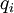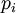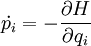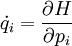# Hamiltonian$H (q,p,t) = \dot{q_i}p_i -L(q,\dot{q},t)$
where$q_i$ are the generalised coordinates,$p_i$ are the canonical momentum, and L is the Lagrangian. Using the Hamiltonian function, the equations of motion can be expressed in the so-called canonical form:$\dot{p_i} = - \frac{\partial H}{\partial q_i}$$\dot{q_i} = \frac{\partial H}{\partial p_i}$